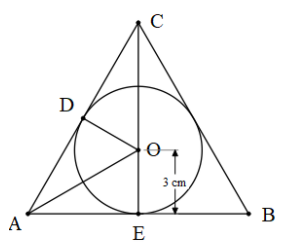The in-radius of an equilateral triangle is of length 3cm, then the length of each of its median is:(a) 12 cm(b) $\dfrac{9}{2}$(c) 4 cm(d) 9 cmVerified
149.1k+ views
Hint: In order to solve this problem, with the help of the properties of the equilateral triangle, we need to identify the right-angle triangle and we can then solve the problem with the help of the trigonometric ratio. The trigonometric ratio used in this question is $\sin x=\dfrac{\text{opposite side}}{\text{hypotenuse}}$ .

Complete step-by-step solution:
In this problem, we are given an equilateral triangle.
And we need to find the length of the median.
We are given the radius of the inscribed circle.
We can understand it better.We can see that length of OE is 3 cm.
We need to find the length of CO.
As all the sides of the equilateral triangle are equal, we can say that CO = OA by symmetry.
WE know that all the angles in an equilateral triangle are ${{60}^{\circ }}$.
In an equilateral triangle, the segment AO acts like an angle bisector, so,
$\angle OAE=\dfrac{60}{2} = {{30}^{\circ }}$
Now, let's consider the triangle OAE.
CE acts like a median, perpendicular bisector, and an angle bisector in an equilateral triangle.
So $\angle OEA={{90}^{\circ }}$
We know that the sum of all angles in a triangle is ${{180}^{\circ }}$.
So, $\angle A+\angle E+\angle O={{180}^{\circ }}$
Solving for $\angle O$ we get,
\begin{align} & 30+90+\angle O={{180}^{\circ }} \\ & \angle O={{60}^{\circ }} \\ \end{align}
Therefore, now we can use the trigonometric ratios.
$\sin x=\dfrac{\text{opposite side}}{\text{hypotenuse}}$
Substituting we get,
$\sin 30=\dfrac{OE}{OA}$
Solving for OA we get,
\begin{align} & \dfrac{1}{2}=\dfrac{3}{OA} \\ & OA=3\times 2=6 \\ \end{align}
Therefore, the length of OA = OC = 6cm.
We need to find the length of CE which is CO + OE.
Substituting we get,
CE = CO + OE = 6 + 3 = 9 cm.
Hence, the correct option is (d).

Note: In this problem, we can directly use the theorem that the centroid dives the median in the ratio of 2: 1. So we can directly get the value of OA = 3 x 2 = 6cm. In this calculation, we need to be careful of which side is twice of which side.